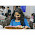## Monday, 21 January 2013

### CBSE Class 10 - Maths - Applications of Trigonometry (MCQs)

Applications of TrigonometryLand Surveyor using Theodolite

(MCQs)

Q1: When a point is observed, the angle formed by the line of sight with the horizontal level where the point being viewed is above the horizontal plane is known as:

(a) angle of triangle
(b) angle of depression
(c) angle of elevation
(d) none of these

Q2: When we raise our hand to look at the object, the angle formed by the line of sight with
horizontal is known as:

(a) obtuse angle
(b) angle of elevation
(c) angle of depression
(d) acute angle

Q3: When we lower our hand to look at the object, the angle formed by the line of sight with
horizontal is known as:

(a) obtuse angle
(b) angle of elevation
(c) angle of depression
(d) acute angle

Q4: When the length of the shadow of a pillar is equal to its height, the elevation at source of
sight is:

(a) 30°
(b) 45°
(c) 60°
(d) 90°

Q5: A pole 10 m high casts a shadow 10 m long on the ground, then the sun’s elevation is?

(a) 60°
(b) 45°
(c) 30°
(d) 90°

Q6: The angle of depression from the top of a tower 12 m high, at a point on the ground is 30°. The distance of the point from the top of the tower is:

(a) 12 m
(b) 4√3 m
(c) 12√3 m
(d) 24 m

Q7: A ladder is 10 m long. It touches a wall at height of 5 m. The angle θ made by it with the horizontal is:

(a) 90°
(b) 60°
(c) 45°
(d) 30°

Q8: If the angle of depression of an object from a 75 m high tower is 30°, then the distance of the object from the base of tower is:

(a) 25√3 m
(b) 50√3 m
(c) 75√3 m
(d) 150 m

Q9: The ratio of the length of a rod and its shadow is 1 :√3. The altitude of the sun is:

(a) 30°
(b) 45°
(c) 60°
(d) 90°

Q10: The tops of two poles of height 10m and 18m are connected with wire. If wire makes an angle of 30° with horizontal, then length of wire is:

(a) 10 m
(b) 12 m
(c) 16 m
(d) 18 m

Available as Online Quiz.

1: (c) angle of elevation
2: (b) angle of elevation
3: (c) angle of depression
4: (b) 45°
5: (b) 45°
6: (b) 4√3 m
7: (d) 30°
8: (c) 75√3 m
9: (a) 30°
10: (c) 16m1.2.